# Kerala SSLC Maths Model Question Paper 2 English Medium

Students can Download Kerala SSLC Maths Model Question Paper 2 English Medium, Kerala SSLC Maths Model Question Papers helps you to revise the complete Kerala State Syllabus and score more marks in your examinations.

## Kerala SSLC Maths Model Question Paper 2 English Medium

Time: 2½ Hours
Total Score: 80 Marks

Instructions

• Give explanations wherever necessary.
• First 15 minutes is Cool-off time. You may use the time to read the questions and plan your answers.
• No need to simplify irrationals like √2, √3, π etc., using approximations unless you are asked to do so.

Answer any three questions from 1 to 4. Each question carries 2 scores. (3 × 2 = 6)

Question 1.
a) Write an arithmetic sequence with 7 as common difference
b) How much should be added with 4th term to get 15th term?
a) 1, 8, 15, ………

Question 2.
In the picture AC, OB meets at point P. ΔBPC is an equilateral triangle.a) Find ∠C
b) Find ∠AOB
a) ∠C = 60°
b) ∠AOB = 2 × 60 = 120°Question 3.
In ΔABC, ∠90°, BC = 15 cm, sin B = 0.35
a) Construct the figure approximately.
b) Find the length of AC.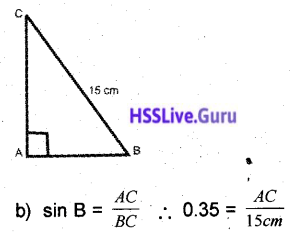AC = 15 × 0.35 = 5.25 cm

Question 4.
The area of a solid sphere is 200 cm2. If we cut and divide it into two hemispheres, what will be the area of the hemisphere?
4πr2 = 200 cm2
πr2 = 50 cm2
3πr2 = 3 × 50 = 150 cm2

Write any 5 questions from 5 to 11. Each question carries 3 marks each. (5 × 3 = 15)

Question 5.
The sides of a rectangle are parallel to x and y-axis. The co-ordinates of opposite vertices are (-2, 3), (6, 9)
a) Find the coordinates of the other two vertices.
b) Find the length of diagonals.
a) (-2, 9), (6, 3)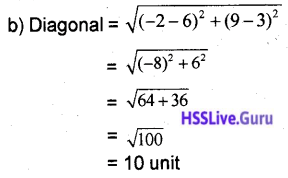Question 6.
A and B are points on a circle of radius 4 cm. O is the centres. ∠AOB = 120°. The tangents from A and B meet at point P.
a) Draw the appropriate figure
b) Find the length of the OP, APQuestion 7.
P(x) = x2 – 8x + 12
a) Find the value of P(2)
b) Write the factors of P(x)
a) P(2) = 22 – 8 × 2 + 12 = 4 – 16 + 12 = 0
b) x2 – 8x + 12 = (x – 6) (x – 2)Question 8.
If 1 + 2 + 3 + ……… + 20 = 210
Find
a) 3 + 6 + 9 + …… + 60
b) 5 + 8 + 11 + ……. + 62
a) 3(1 + 2 + 3 + …. + 20) = 3 × 210 = 630
b) 3 + 2 + 6 + 2 + 9 + 2 + ……….. + 60 + 2
= (3 + 6 + 9 + ……. 60) + (2 + 2 + …… 20 times)
= (3 + 210 + 2 × 20
= 630 + 40
= 670

Question 9.
One angle in a rhombus is 120°. One side = 6 cm. To find the length of its diagonal.
a) If ∠PAB = x°, then what will be ∠PBA
b) Then prove that ΔPAB is equilateral.
Half of diagonals = $$\frac{6}{\sqrt{3}}$$ = 2√3, 4√3
Diagonals = 4√3, 8√3 cm

Question 10.
The base edge of a square prism is 16 cm and height 15 cm. From this a square pyramid of maximum height is cut off
a) What is its maximum height?
b) Find slant height
c) Find lateral surface area?
a) h = 15 cm
b) $$\sqrt{15^{2}+8^{2}}=\sqrt{289}$$ = 17 cm
c) 2al = 2 × 16 × 17 = 544 cm2

Question 11.
a) What is the slope of the life 3x – 2y – 6 =0
b) Write the coordinates on which the line cuts x and y axis?
a) slope = $$-\left(\frac{3}{-2}\right)=3 / 2$$
b) x axis : y = 0
3x – 2 × 0.6 = 0
3x = 6
x = 6/3 = 2
∴ (2, 0)
y axis : x = 0
3 × 0 – 2y – 6 = 0
y = $$\frac{6}{-2}$$ = -3
∴ (0, -3)Answer any 7 questions from 12 to 21. Each question carries 4 score. (7 × 4 = 28)

Question 12.
A box contains some paper pieces in which the numbers from 1 to 20 are written. If we take one without looking.
a) What is the probability of it being a prime number?
b) Probability of its being a perfect square.
c) What is the probability of being prime or perfect square?
a) $$\frac{8}{20}=\frac{2}{5}$$
b) $$\frac{4}{20}=\frac{1}{5}$$
c) $$\frac{12}{20}=\frac{3}{5}$$

Question 13.
The sum of first n terms in an arithmetic sequence is $$\frac{5}{2} n^{2}+\frac{3}{2} n$$
a) What is the first term?
b) Find the common difference
c) Find the sum of first 12 terms.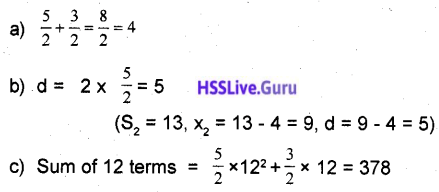Question 14.
In a square pyramid, all edges are of the same length. Its height is 12√2 cm.
a) What is the length of its base edge?
b) Find volume.
a) Base edge
b) Lateral edge
e) Slant height (l)Question 15.
P(x) = kx2 – 6x – 5. The reminder got when p(x) is divided by 2 is 3.
a) Find P(2).
b) Find the value of 4.
c) How much should be added to P(x), for (x + 2) to be a factor of P(x)?
a) P(2) = 3
b) K × 22 – 6 × 2 – 5 = 3
4K – 12 – 5 = 3
4K = 20
K = $$\frac{20}{4}$$ = 5
c) P(-2)= 5 × (-2)2 – 6 × -2 – 5 = 20 + 12 – 5 = 27
∴ K = added – 27.Question 16.
Consider the lines 2x + 3y = 12, x – 2y = -1
a) Find the coordinate at which both the lines meet.
b) Form the equation of the line which passes through this point and the slope ½.Question 17.
In figure 2 circles meet at the points Q, R. PA = PB
a) Prove PA × PC = PB × PD
b) Prove ∠C = ∠D
c) Prove ABDC is a cyclic quadrilateral.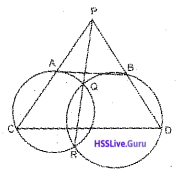a) PA × PC = PQ × PR = PB × PD
∴ PA × PC = PB × PB
b) If PA = PB, PC = PD
ΔPCD is Isosceles
∠C = ∠D
c) ∴ ABCD is an isoscelless trapezium
∴ ABDC is cyclic

Question 18.
In the figure, the circle with O as center is the circum circle of ∆ABC. ∠A = 50°, ∠B = 65°. AB = 8 cm.
a) Find ∠C.
b) Find the diameter.
c) Find AC, BC. (sin 50° = 0.76, sin 65° = 0.90)∠C = 65°
b) $$2 r=\frac{A B}{\sin C}, 2 r=\frac{8}{0.9}$$
Diameter = 8.89
b) AC = 8.89 × sin 65 = 8.89 × 0.9 = 8 cm or isoscelles triangle.
So AC = AB
BC = 8.89 × sin 50 = 8.89 × 0.76 = 6.75 cmQuestion 19.
The perimeter of a right triangle is 40 cm. The hypotenuse is 17 cm.
a) What is the sum of the other two sides?
b) If one side is x what is the other side?
c) Find the length of both sides.
a) 23
b) 23 – x
c) x2 + (23 – x)2 = 172
x2 + x2 – 46x + 529 = 289
x2 – 23x + 120 = 0
Value of x = 15 cm.
Sides = 8 cm, 15 cm, 17 cm

Question 20.
P(x) = 2x3 – 7x2 + ax + b
a) If x – 1, x – 3 are factor of P(x) find P(1), P(3)
b) Find the value of a, b.
c) Find the third factor of P(x)
a) P(1) = -5 + a + b = 0
P(3) = -9 + 3a + b = 0
b)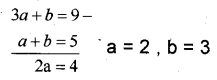c) 2x3 – 7x2 + 2x + 3
3rd factor (2x + 1)

Question 21.
From a hemisphere, a square pyramid of maximum size is cut off. Its height is 9 cm.
a) What is the radius of the hemisphere?
b) What is the base edge of the square pyramid?
c) Find the volume of the square pyramid.
a) 9 cm
b) $$\frac{18}{\sqrt{2}}$$ or 9√2 diameter is the diagonal of square.
c) Volume = $$\frac{1}{3} \times(9 \sqrt{2})^{2} \times 9$$ = 486 cm3Answer any 5 questions from 22 to 28. Each question carries 5 marks. (5 × 5 = 25)

Question 22.
Consider the arithmetic sequence 4, 7, 10, 13, ……..
a) Write its algebraic expression.
b) Is 169 a term of this sequence?
c) Prove that the square of any term of this sequence is also a term in this arithmetic sequence.
a) 3n + 1
b) Yes 169 = 3 × 56 + 1
c) (3n + 1 )2 = 9n2 + 6n + 1
If we divde with 3 remainder is 1.
Square of any term is also a term in this sequence.

Question 23.
In ΔABC. AB = 6 cm, AC = 7 cm, ∠A = 70°
a) Draw the triangle and draw a rectangle of the same area.
b) Construct a square of equal area to the triangle.
a) Draw the triangle, then draw its height, draw its perpendicular bisector. Taking the half of height and base complete the rectangle.
b) Then extend its breadth along with its length. Find its midpoint complete the semicircle. Draw a perpendicular from the end of the length. Taking that long to complete a square.

Question 24.
The area of a cone is 628 cm2 slant height is 17 cm.
b) Find volume.
a) πr (R + r) = 628
⇒ πr (17 + r) = 628
⇒ r(17 + r) = $$\frac{628}{3.14}$$ = 200
⇒ r2 + 17r = 200
⇒ r2 + 17r – 200 = 0
⇒ r = 3 and r = -25
Height = $$\sqrt{17^{2}-8^{2}}$$ = 15 cm.
b) Volume = $$\frac{1}{3} \pi \times 8^{2} \times 15=320 \pi \mathrm{cm}^{3}$$Question 25.
The daily wages of workers in a factory is given in the table.

 Daily wage No.of workers 200 – 300 3 300 – 400 5 400 – 500 6 500 – 600 8 600 – 700 10 700 – 800 7 800 – 900 5 900 – 1000 3

a) If the workes are arranged according to their wages. Which members comes as the median?
b) What is the wage of the 23rd member?
c) Find the wage which comes as the median.
300 – 3
400 – 8
500 – 14
600 – 22
700 – 32
800 – 39
900 – 44
1000 – 47
a) Coolie of 24th Man
b) 600 + 5 = 605
b) 605 + 10 = 615

Question 26.
Construct an equilateral triangle of side 6 cm and draw its incircle.
Construction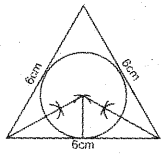Question 27.
A circle having the centre (3, 4) passes through the origin.
b) It one point on the circle is (x, y) find the relation between x, y
c) Check whether (-2, 1) a point on the circle?
a) $$r=\sqrt{3^{2}+4^{2}}=5 \text { unit }$$
b) $$r=\sqrt{(x-3)^{2}+(y-4)^{2}}$$
⇒ (x – 3)2 + (y – 4)2 = 52
⇒ x2 + y2 – 6x – 8y = 0
c) (-2)2 + 12 – 6 × (-2) – 8 × 1
= 4 + 1 + 12 – 8 ≠ 0
-2, 1 is not a point on circleQuestion 28.
The chord BD is perpendicular to the diameter AC. Find the angles.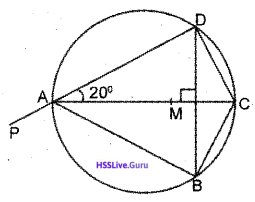a) ∠BAC
b) ∠BCD
d)∠CDM
e) ∠BAP
a) ∠BAC = 20°
b) ∠BCD = 140°
d) ∠CDM = 70°
e) ∠BAP = 140°

Read the mathematical concept carefully and answer the following question. (6 × 1 = 6)

Question 29.
An arrangement of data in rows and columns. Using numbers is known as a matrix. Usually, the matrix is represented by English capital letters.
If a matrix contains 2 raw and 3 columns has an order of 2 × 3. It can be read as ‘2 by 3’ and is represented by 2 × 3. If you say an order say the number of rows, then namber of columns. For example
$$A=\left[\begin{array}{ll} 1 & 2 \\ 4 & 5 \\ 7 & 8 \end{array}\right]$$ order of this matrix is 2 × 3
If the number of rows, and number of columns are different. It is known as rectangular matrix. It both are same then it is square matrix. If the order of matrices are same men it can be add, subtract, ….
for example $$A=\left[\begin{array}{ll} 2 & 4 \\ 6 & 8 \end{array}\right] B=\left[\begin{array}{ll} 3 & 5 \\ 7 & 9 \end{array}\right]$$
$$A+B=\left[\begin{array}{ll} 2+3 & 4+5 \\ 6+7 & 8+9 \end{array}\right]=\left[\begin{array}{cc} 5 & 9 \\ 13 & 17 \end{array}\right]$$
we can do the subtraction also like this.
a)
$$A=\left[\begin{array}{lll} 1 & 2 & 3 \\ 4 & 5 & 6 \end{array}\right]$$
i) Write the order of A
ii) Which type of matrix is A?
b) If B has ‘m’ rows as ‘n’ columns write the order of B
c) If $$C=\left[\begin{array}{lll} 1 & 2 & 3 \\ 4 & 5 & 6 \end{array}\right], D=\left[\begin{array}{ccc} 7 & 8 & 9 \\ 10 & 11 & -12 \end{array}\right]$$
i) Find C + D
ii) Find D – C
d) If the matrix X has an order 4 × 5, y has an order 4 × 5 what will be the order of x + y
c) $$\mathrm{C}+\mathrm{D}\left[\begin{array}{l} 8,10,12 \\ 14,16,18 \end{array}\right] ; \mathrm{C}+\mathrm{D}=\left[\begin{array}{l} 6,6,6 \\ 6,6,6 \end{array}\right]$$